# 1 Euclidean transformations

On the set of all real points of the space ${}_{\infty }{\mathbf{E}}^{n}\left(R\right)$ with defined homogeneous coordinate system, there is defined a group of Euclidean (metric) transformations called isometries - isometric mappings, or congruences. Metric transformations of the Euclidean space keep invariant (in addition to incidence) the size of line segments, which is the metric of the space, therefore the size of geometric figures does not change under these transformations. Image of a geometric figure (original) under a metric transformation is geometric figure congruent to the original.

Metric transformations of the space are: identity, symmetry about a plane, symmetry about a line - axial symmetry, symmetry about a point - central symmetry, revolution about a point or a line, translation, translated symmetry about a line or a plane, revolved symmetry about a plane, helical movement.

Metric transformations are analytically represented by regular square matrices of rank 4, while determinant of the matrix equals $±1$, which means ${|{\mathrm{T}}_{\mathrm{M}}|}^{2}=1$ and the matrix is in the form

 ${\mathrm{T}}_{\mathrm{M}}=\left(\begin{array}{cccc}{a}_{11}& {a}_{12}& ...& 0\\ {a}_{21}& {a}_{22}& ...& 0\\ .& .& .& 0\\ {a}_{n+1,1}& {a}_{n+1,2}& ...& 1\end{array}\right)$

Entries ${a}_{\mathrm{ij}}\phantom{\rule{2mm}{2mm}}$of matrix ${\mathrm{T}}_{\mathrm{M}}$ for $i=1,2,3,4$ and $j=1,2,3$ are coefficients of separate Euclidean metric transformations and they have a specific geometric interpretation.

${a}_{11},\phantom{\rule{2mm}{2mm}}{a}_{22},\phantom{\rule{2mm}{2mm}}...,\phantom{\rule{2mm}{2mm}}{a}_{nn}\phantom{\rule{2mm}{2mm}}$are nonzero scales on the coordinate axes x, y, z, ... respectively,

${a}_{n+1,1},\phantom{\rule{2mm}{2mm}}{a}_{n+1,2},\phantom{\rule{2mm}{2mm}}...,\phantom{\rule{2mm}{2mm}}{a}_{n+1,n}$ are coordinates of the translation vector

## 1.1 Transformations of the Euclidean plane

In the two-dimensional extended Euclidean space ${}_{\infty }{\mathbf{E}}^{2}\left(R\right)$ with the defined homogeneous coordinate system, a group of Euclidean metric transformations can be determined on the subset of all real points ${\mathbf{E}}^{2}\left(R\right)$. Any real point $A=$$\left(x,y,1\right)$ in the subspace can be attached its image $A\text{'}=\left(x\text{'},y\text{'},1\right)$, while the relation of coordinates can be expressed in the following way

 $A\text{'}=A.{\mathrm{T}}_{\mathrm{M}}$

where ${\mathrm{T}}_{\mathrm{M}}\phantom{\rule{mediummathspace}{0.2em}}$is the regular square matrix of rank 3.

We recognize the following metric transformations of the plane: identity, symmetry about a point - central symmetry, symmetry about a line - axial symmetry, revolution about a point, translation, shifted axial symmetry.

### 1.1.1 Symmetry about a point

Let the centre of symmetry be the origin O, then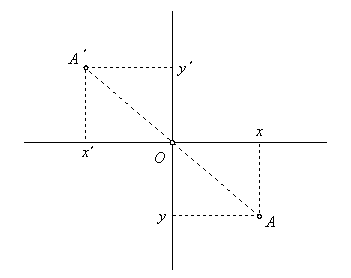Figure 1.1: Central symmetry

Equations and matrix of central symmetry

 $x\text{'}=-x$
 $y\text{'}=-y$
 ${\mathrm{T}}_{\mathrm{M}}=\left(\begin{array}{ccc}-1& 0& 0\\ 0& -1& 0\\ 0& 0& 1\end{array}\right)$

### 1.1.2 Symmetry about a line

Let the axis of symmetry be in the coordinate axis x, then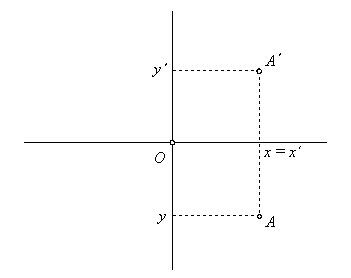Figure 1.2: Axial symmetry

Equations and matrix of axial symmetry

 $x\text{'}=x$
 $y\text{'}=-y$
 ${\mathrm{T}}_{\mathrm{M}}=\left(\begin{array}{ccc}1& 0& 0\\ 0& -1& 0\\ 0& 0& 1\end{array}\right)$

Let the axis of symmetry be in the coordinate axis y, then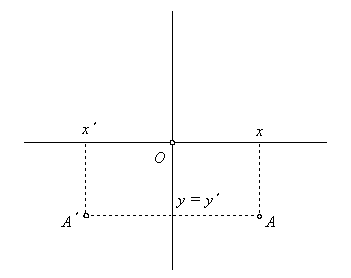Figure 1.3: Axial symmetry

Equations and matrix of axial symmetry

 $x\text{'}=-x$
 $y\text{'}=y$
 ${\mathrm{T}}_{\mathrm{M}}=\left(\begin{array}{ccc}-1& 0& 0\\ 0& 1& 0\\ 0& 0& 1\end{array}\right)$

### 1.1.3 Revolution about a point

Let the centre of revolution be in the origin O, then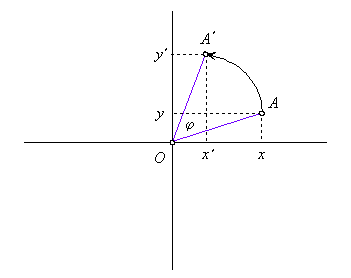Figure 1.4: Revolution about centre

Equations and matrix of revolution about centre

 $x\text{'}=x\phantom{\rule{2mm}{2mm}}\mathrm{cos}\phantom{\rule{2mm}{2mm}}\varphi -y\phantom{\rule{mediummathspace}{0.2em}}\mathrm{sin}\phantom{\rule{mediummathspace}{0.2em}}\varphi$
 $y\text{'}=x\phantom{\rule{2mm}{2mm}}\mathrm{sin}\phantom{\rule{2mm}{2mm}}\varphi +y\phantom{\rule{mediummathspace}{0.2em}}\mathrm{cos}\phantom{\rule{mediummathspace}{0.2em}}\varphi$

 ${\mathrm{T}}_{\mathrm{M}}=\left(\begin{array}{ccc}\mathrm{cos}\phantom{\rule{mediummathspace}{0.2em}}\varphi & \mathrm{sin}\phantom{\rule{mediummathspace}{0.2em}}\varphi & 0\\ -\mathrm{sin}\phantom{\rule{mediummathspace}{0.2em}}\varphi & \mathrm{cos}\phantom{\rule{mediummathspace}{0.2em}}\varphi & 0\\ 0& 0& 1\end{array}\right)$

### 1.1.4 Translation

Let the translation vector be given by homogeneous coordinates $\mathbf{a}=\left(m,n,0\right)$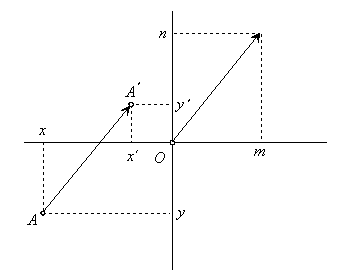Figure 1.5: Translation by vector

Equations and matrix of translation

 $x\text{'}=x+m$
 $y\text{'}=y+n$
 ${\mathrm{T}}_{M}=\left(\begin{array}{ccc}1& 0& 0\\ 0& 1& 0\\ m& n& 1\end{array}\right)$

### 1.1.5 Translated symmetry about a line

Translated symmetry about a line is transformation composed from symmetry about a line and translation in direction perpendicular to the axis of symmetry.

Let the translation in the direction perpendicular to the axis of symmetry in coordinate axis x be given by vector $\left(0,c,0\right)$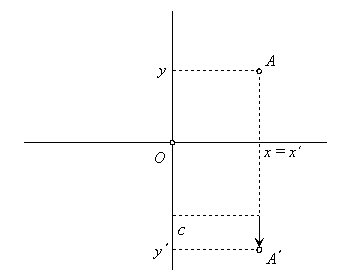Figure 1.6: Translated axial symmetry

Equations and matrix of translated axial symmetry

 $x\text{'}=x$
 $y\text{'}=-y+c$
 ${T}_{M}=\left(\begin{array}{ccc}1& 0& 0\\ 0& -1& 0\\ 0& c& 1\end{array}\right)$